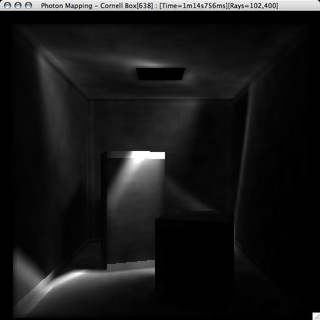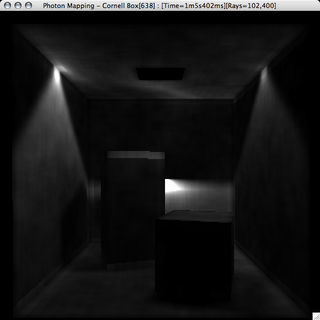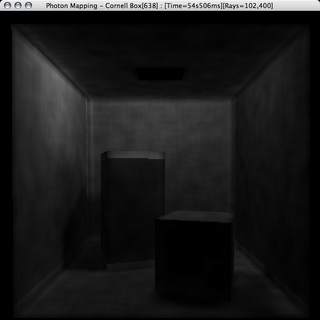# Success! Atleast with GIThis has been an incredibly annoying project. Granted it stems from the fact that I didn't have all the resources I needed as far as the minute detials behind programming the ray tracer. I didn't have Jensen's book Realistic Image Synthesis Using Photon Mapping, which supposedly is a pretty decent book, and most importantly has functioning code in the back. The biggest problem I had was that everyone explained the generalities of the algorithem, but not the depths of the implementation, and no one posted their code up, which I will do on the completion of my project, in hopes that someone else won't go through what I did.

There were a fair number of more specfic problems with my implementation of a photon-mapper. One of which were my photon lights, and my seemingly inability to get the photons to cast in the correct directions. This has to be a side effect of the algorithem used for picking a random-point on a sphere, but as why I had so much trouble is unbeknowst to me. Here are three images showing a grays-scale direct visualization of the photon map, i.e. the bright the area, the more photons landed neat there.

This first picture is from my first method of picking a point on a hemisphere to aim the photons from the light. I have no idea what is up with the focusing and the voids, it completely baffles me.Here is a slightly better image, the photons are shooting in more reasonible directions in reasonibl concentrations, but still its not good enough, things just are quite right.

Finally, after moving to an entirely new method of picking a point on a sphere I get this, which looks great!For those of you who care, here are my methods for generating points on a unit sphere. This first one is just not working for me:

```        double x1 = 0, x2 = 0, x12 = 0, x22 = 0;
do
{
x1 = RAND.uniform( -1, 1 );
x2 = RAND.uniform( -1, 1 );
x12 = x1 * x1;
x22 = x2 * x2;
}
while( x12 + x22 >= 1 );

double x = 2 * x1 * Math.sqrt( 1 - x12 - x22 );
double y = 2 * x2 * Math.sqrt( 1 - x12 - x22 );
double z = 1 - 2 * ( x1 + x2 );

// Stupid co-ordinate frame differences
Vector3d vec = new Vector3d( x, z, y );
return vec;
```

This second one works great for me, so its the one I'm using now:

```        double z = randGen.uniform( -1, 1 );
double t = randGen.uniform( 0, 2.0 * Math.PI );
double w = Math.sqrt( 1 - z * z );
double x = w * Math.cos( t );
double y = w * Math.sin( t );
Vector3d vec = new Vector3d( x, y, z );

// If it is on the other side of the normal, negate the point
if( normal.dot( vec ) < 0 )
{
vec.negate();
}

return vec;```